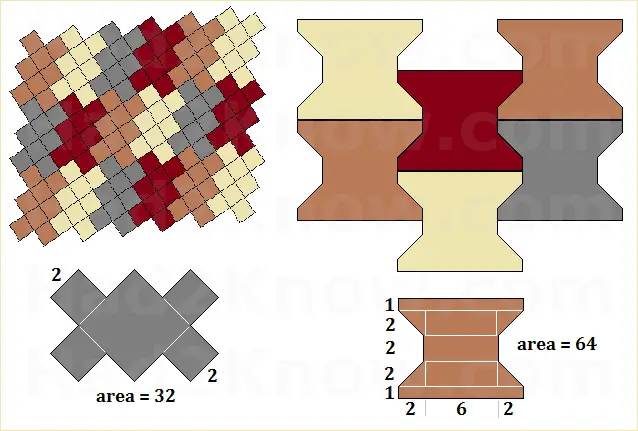# How to Figure the Number of Interlocking Bricks Needed

When you pave with interlocking bricks, you don't need to apply mortar between the blocks. The shape of the brick is what keeps it in place so that it does not slide against adjacent bricks. One of the tricky things about covering an area with non-rectangular tessellating bricks is figuring out how many you need. Luckily, all you need is simple geometry and arithmetic to solve the problem.

The first step is to determine the area of the brick's face in square inches or cm2. Since interlocking bricks are not perfect rectangles, you must partition the shape into smaller rectangles and triangles. After you find the area of each smaller rectangular and triangular region, add them up to obtain the total area of the brick face.Next, calculate the area of the patio, driveway, or other surface to be paved (in square inches or cm2).

Divide the area of the patio by the brick face area. This is the number of bricks you need to cover the space.

Of course, depending on the shape of the interlocking bricks and the tessellation pattern, you may need more bricks to account for partials along the border. To estimate the number of extra bricks you should buy, divide the perimeter of the paved area by the longest length you can measure across the face of the brick.

Example: You are paving with a type of brick whose area is 22 square inches. The area you need to pave with the bricks is 108 inches wide by 216 inches long. If you measure across the face of the brick, the longest length is about 8 inches.

First, the area of the landing is (108)(216) = 23328 square inches. Since 23328/22 = about 1061, you need at least that many bricks to pave the surface.

The perimeter of the surface is 108 + 108 + 216 + 216 = 648 inches. Since 648/8 = 81, you should buy at least 81 more bricks to be on the safe side.